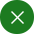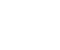Search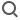English
Application
Application of Lock - in Amplifier in TDLAS Technology

Tunable Diode Laser Absorption Spectroscopy (TDLAS) technology is a detection technology to measure gas component concentrations and temperature fields, or even velocity fields in industrial applications. TDLAS is based on Beer-Lambert's law.  When the laser passes through the gaseous molecular medium,  a portion of the  laser will be absorbed as long as its wavelength is equal to the level transition of any one of the molecules. The relationship between the outgoing light intensity and incident light intensity is as follows: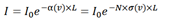Where, α(cm-1 is the absorption coefficient of unit length medium;  L (cm) is the effective absorption length; N (cm-3) is the number of molecules per unit volume (that is concentration); σ(cm-2) is the molecular absorption cross-section. After frequency modulation, and va / v0 << 1, the above equation is written as: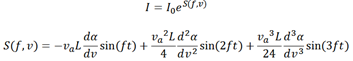From the above equation, it can be seen that the molecule absorbs high-order components of the laser.  In the signal detection path, we use phase-locked amplification technology to achieve the second harmonic detection of the modulated signal and then get  the first, second or third derivative of Gaussian function respectively at 1f, 2f or 3f frequency.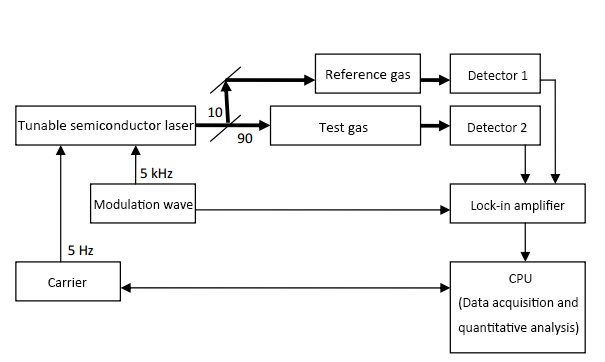In this laser phase-locked system, the most crucial part is the realization of dual-channel detection of test gas and reference gas or even three-channel detection of test gas, reference gas and laser at the same time by lock-in amplifier. OE1022 can successfully solve this problem due to its multiple harmonic measurement function.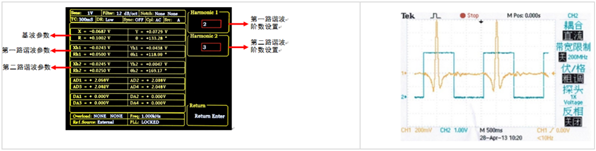Apply for probation